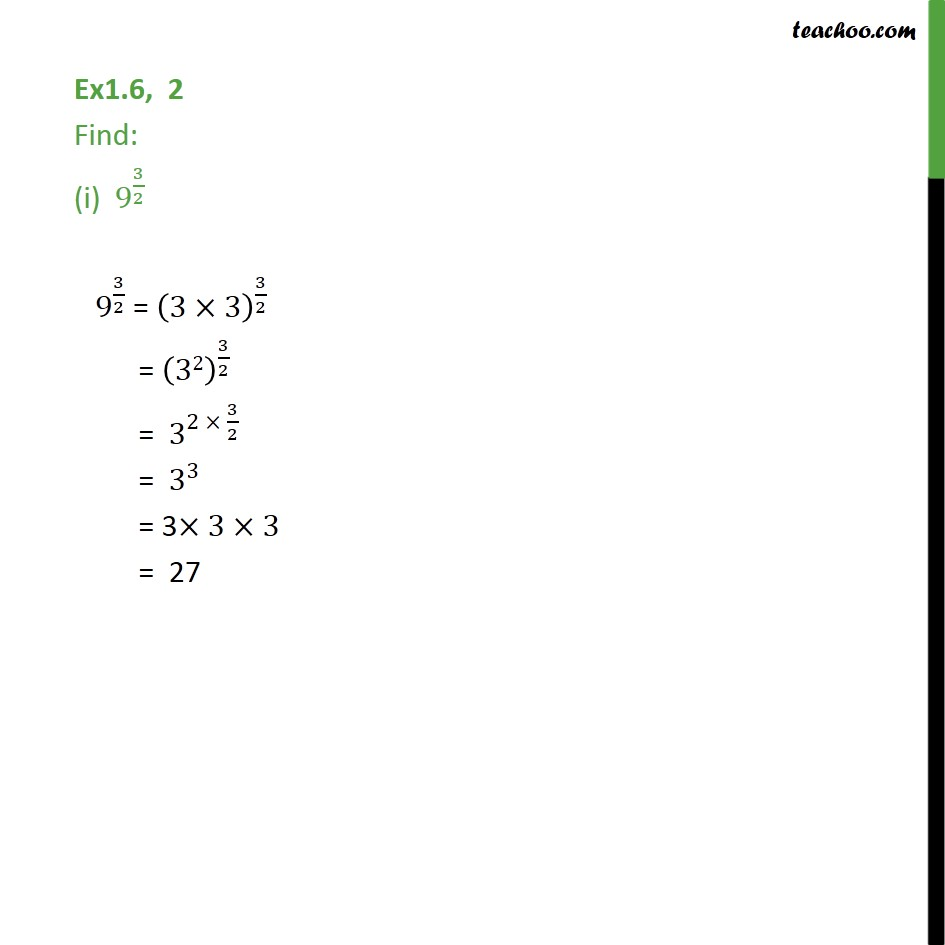1. Chapter 1 Class 9 Number Systems (Term 1)
2. Concept wise
3. Laws of exponents

Transcript

Ex1.6, 2 Find: (i) 9^(3/2) 〖 9〗^(3/2) = (3×3)^(3/2) = (32)^(3/2) = 〖 3〗^(2 × 3/2) = 〖 3〗^3 = 3×3×3 = 27